# The Cantitruncated 120-cell

The cantitruncated 120-cell is a beautiful uniform polytope from the 120-cell family of uniform polychora.

It is bounded by 1920 cells (120 great rhombicosidodecahedra, 1200 triangular prisms, 600 truncated tetrahedra), 9120 polygons (2400 triangles, 3600 squares, 2400 hexagons, 720 decagons), 14400 edges, and 7200 vertices. The above image shows its perspective projection into 3D, centered on a great rhombicosidodecahedron. For clarity, only facets facing the 4D viewpoint are included; facets lying on the far side of the polytope have been culled.

The cantitruncated 120-cell may be constructed by expanding the decagonal ridges of the truncated 120-cell outwards radially, which causes the truncated dodecahedra to turn into great rhombicosidodecahedra, the tetrahedra to turn into truncated tetrahedra, and introduces gaps which can be filled in by triangular prisms.

## Structure

We shall explore the structure of the cantitruncated 120-cell using its parallel projections into 3D, centered on a great rhombicosidodecahedron.

### First Layer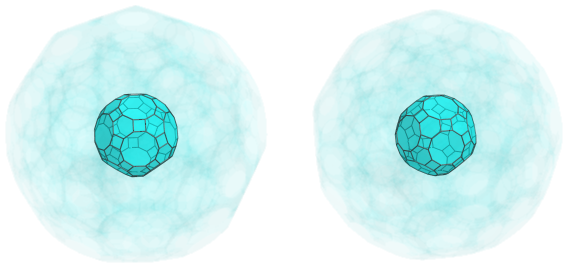The above image shows the nearest cell to the 4D viewpoint, a great rhombicosidodecahedron. For clarity, we have rendered all the other cells in a light transparent color.

### Second Layer

Surrounding this nearest cell are 30 triangular prisms that touch this cell at its square faces: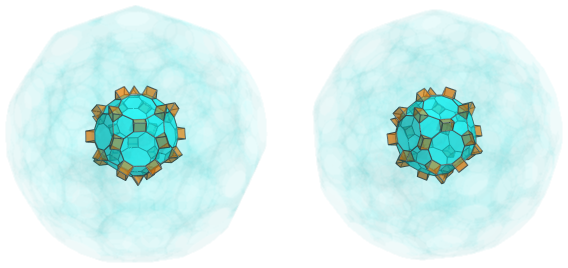There are also 20 truncated tetrahedra that touch the nearest cell at its hexagonal faces: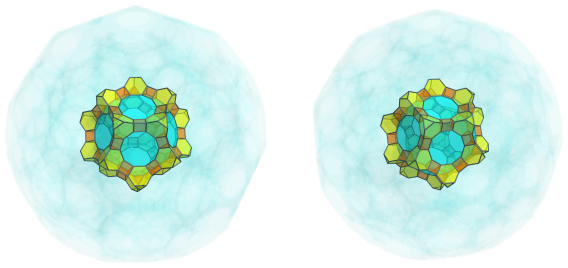These two sets of cells form an alternating pattern around each decagonal face of the nearest cell. This pattern is extended by another 20 triangular prisms that sit atop the truncated tetrahedra: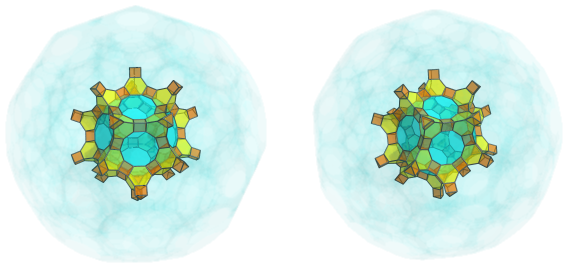And continues with 20 truncated tetrahedra on the other end: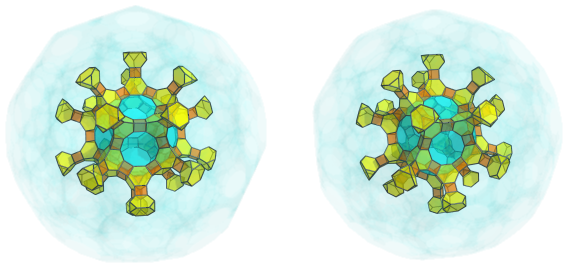These alternating cells form a framework into which 12 great rhombicosidodecahedra, joined to the decagonal faces of the nearest cell, are fitted: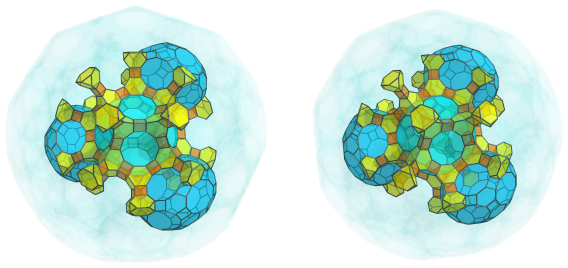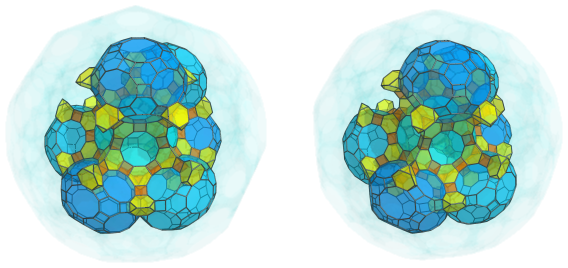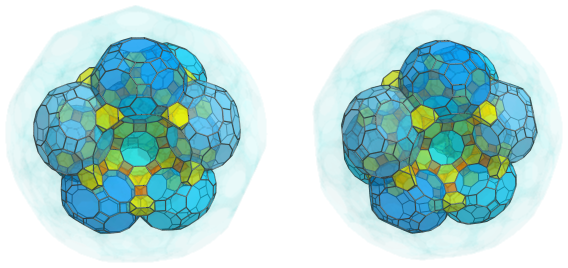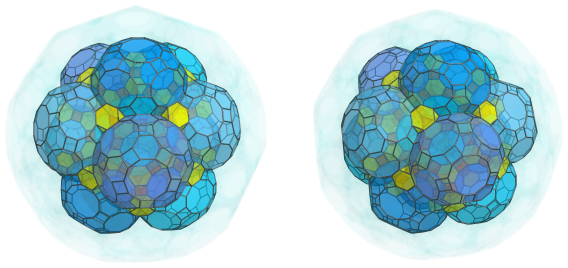### Third Layer

The alternating pattern of triangular prisms and truncated tetrahedra continues with 60 more triangular prisms joined to the visible truncated tetrahedra: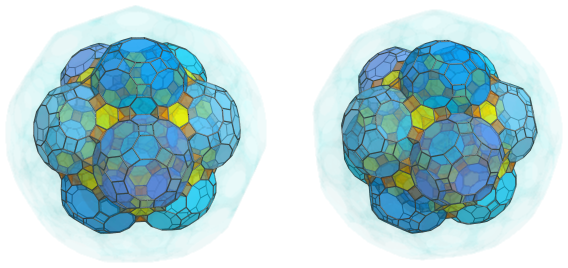And 30 more truncated tetrahedra bridging these triangular prisms: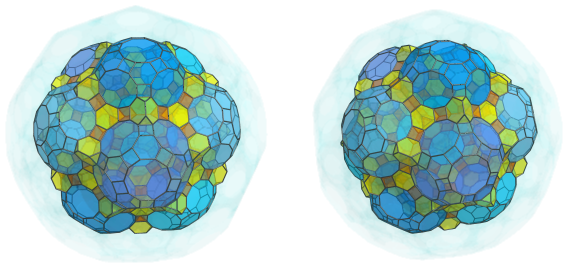And yet another 60 triangular prisms extending from these: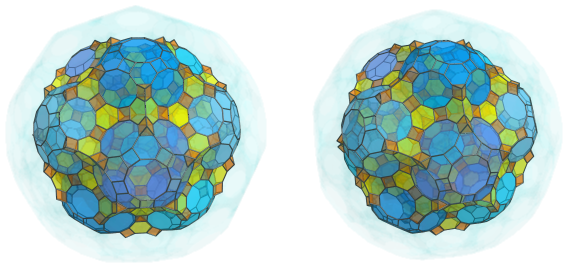Which lead to 60 more truncated tetrahedra: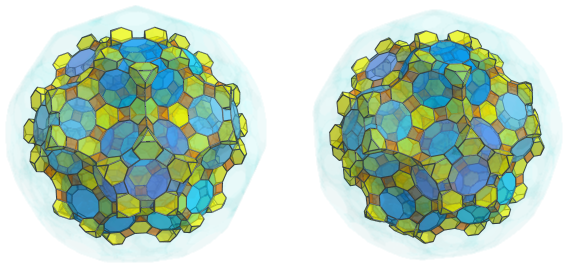Which are, in turn, bridged by another 60 triangular prisms: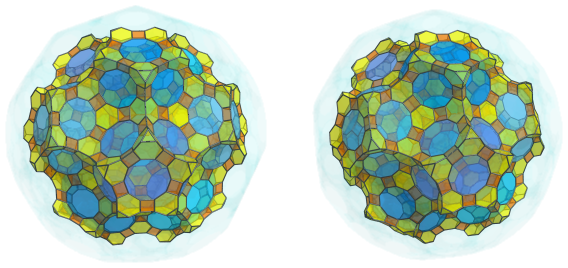Into this network of alternating triangular prisms and truncated tetrahedra another 20 great rhombicosidodecahedra are fitted: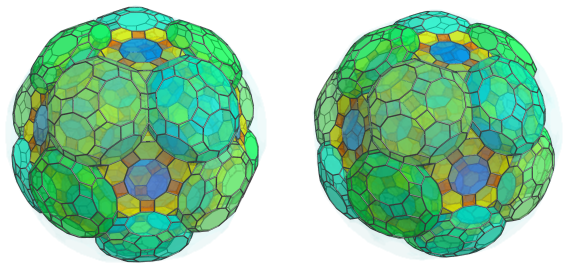### Fourth Layer

The network of alternating truncated tetrahedra and triangular prisms continues with 60 more triangular prisms nestled between adjacent pairs of great rhombicosidodecahedra: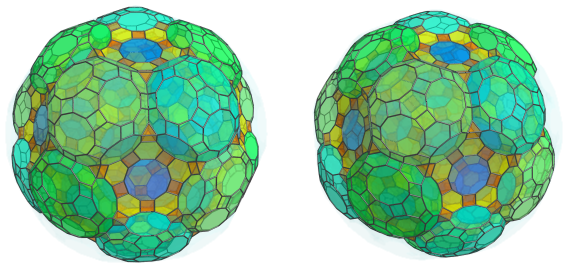They are, of course, topped by 60 truncated tetrahedra, continuing in the pervasive pattern we've seen so far: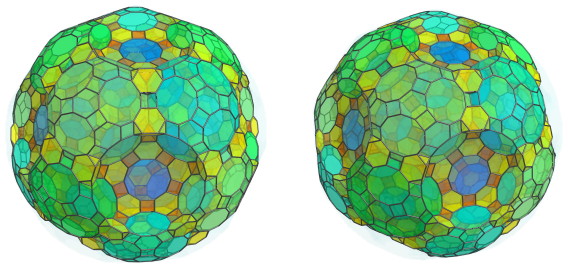These truncated tetrahedra, as expected, are bridged by 30 more triangular prisms: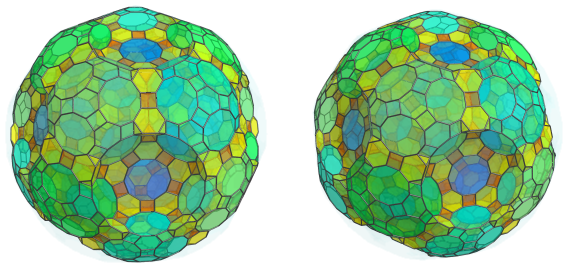The other triangular faces of the truncated tetrahedra are also joined to more triangular prisms, 120 in total: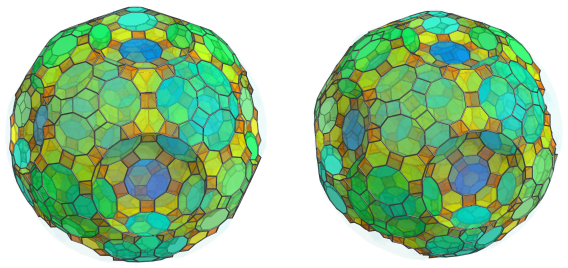These triangular prisms meet at another 60 truncated tetrahedra: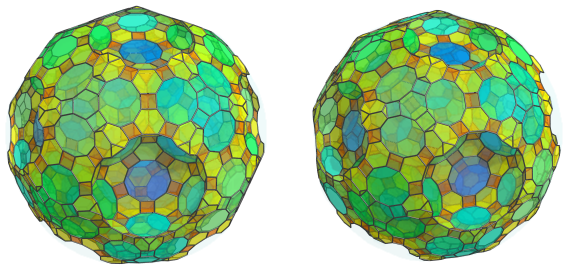Emanating from these truncated tetrahedra are 60 more triangular prisms: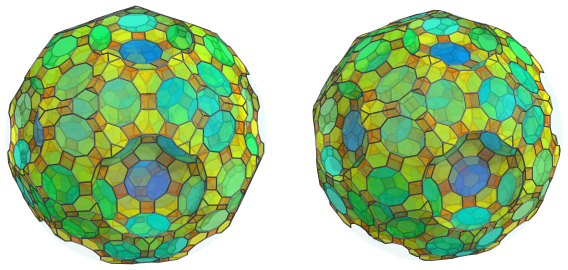These triangular prisms converge on 20 truncated tetrahedra: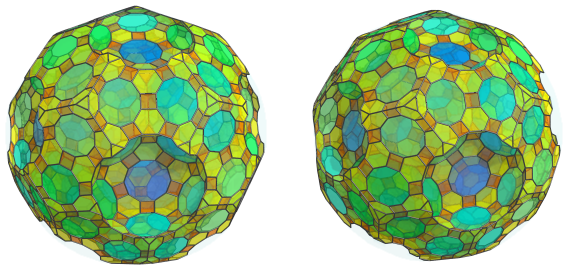The obvious bowl-shaped depressions are filled in by 12 more great rhombicosidodecahedra: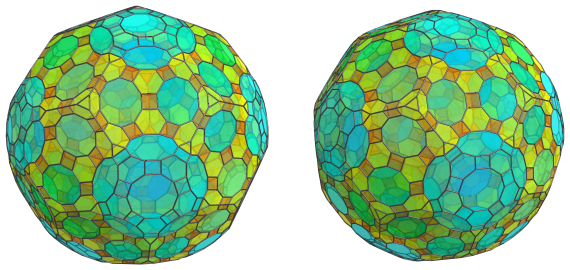Lying against these great rhombicosidodecahedra and the previous truncated tetrahedra are another 60 triangular prisms: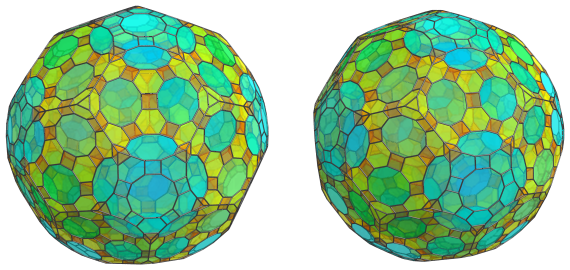These are all the cells that lie on the near side of the cantitruncated 120-cell. The pattern of cells continues past this point on its limb, or “equator”.

### The Equator

There are 30 great rhombicosidodecahedra lying on the equator of the cantitruncated 120-cell, shown below: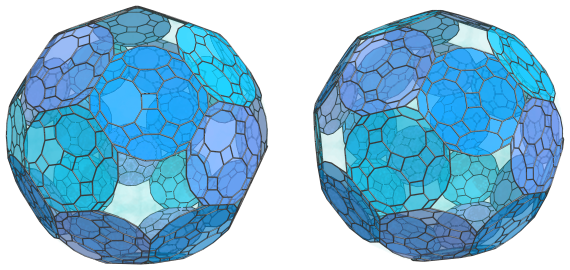For clarity, we have omitted the cells on the near side that we have seen before.

These cells appear flattened into irregular dodecagons because they are at a 90° angle to the 4D viewpoint. In 4D, they are perfectly uniform great rhombicosidodecahedra.

The tiny triangular gaps nestled between these cells are where 20 triangular prisms are located, forming the vertices of a virtual dodecahedron: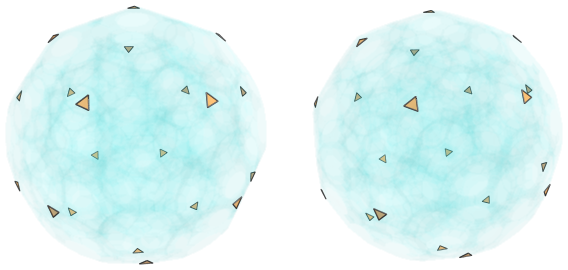We have omitted the previous great rhombicosidodecahedra so that the triangular prisms are more easily discernible. They have been foreshortened into triangles because they are seen from a 90° angle. In 4D, they are perfectly uniform triangular prisms.

Here are both sets of cells together: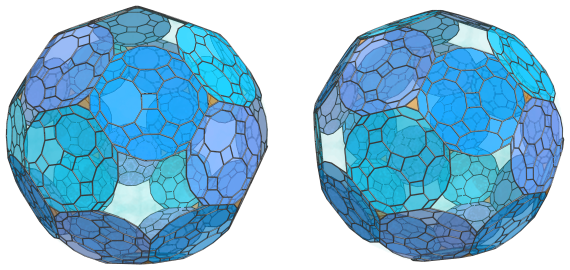These aren't the only triangular prisms on the equator; there are 60 others, also touching the great rhombicosidodecahedra, but in a different orientation: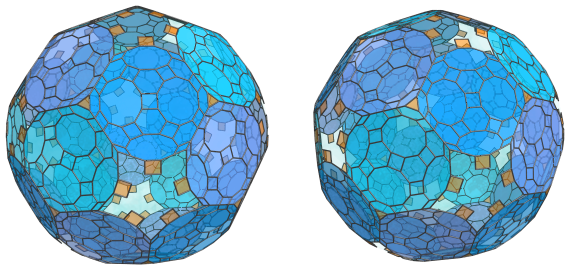These triangular prisms have been foreshortened into rectangles rather than triangles, because they are oriented differently from the previous triangular prisms, even though both are being seen at a 90° angle.

They alternate with 60 truncated tetrahedra, shown next: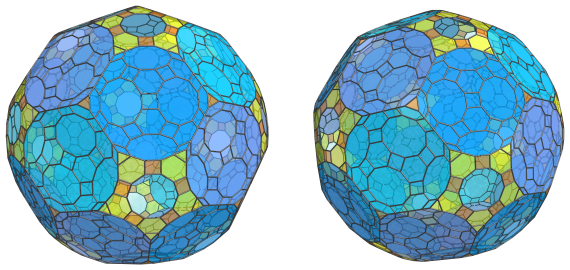These truncated tetrahedra appear as irregular pentagons due to their being seen from a 90° angle, but in reality they are perfectly uniform truncated tetrahedra.

These are all the cells that lie on the equator of the cantitruncated 120-cell. Past this point, we get to its far side. The remaining 12 decagonal gaps are not the images of any cells; they are where 12 great rhombicosidodecahedral cells on the near side touch their counterparts on the far side. The arrangement of cells on the far side mirrors that of the near side, repeating in reverse order until it ultimately converges on the antipodal great rhombicosidodecahedron.

### Summary

The cell counts of each layer of the cantitruncated 120-cell are summarized in the following table:

Region Layer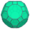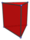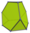Near side 1 1 0 0
2 12 30 + 20 = 50 20 + 20 = 40
3 20 60 + 60 + 60 = 180 30 + 60 = 90
4 12 60 + 30 + 120 + 60 + 60 = 330 60 + 60 + 20 = 140
Subtotal 45 560 270
Equator 30 20 + 60 = 80 60
Far side 4 12 330 140
3 20 180 90
2 12 50 40
1 1 0 0
Subtotal 45 560 270
Grand total 120 1200 600

## Coordinates

The coordinates of the cantitruncated 120-cell, centered on the origin and with edge length 2, are all permutations of coordinates and changes of sign of:

• (1, 1, 1+4φ, 5+10φ)
• (1+3φ, 3φ2, 3+7φ, 3+7φ)
• 4, φ4, 2+7φ, 4+7φ)

as well as even permutations of coordinates and all changes of sign of:

 (0, 1, 6+7φ, 3+7φ) (0, 1, 6+9φ, 1+5φ) (0, φ2, φ6, 2+7φ) (0, φ2, 7+8φ, 2+5φ) (0, 2φ, 6+8φ, 2+6φ) (1, 4+5φ, 2φ4, 5φ2) (1, 2, 5+7φ, 4+7φ) (1, 2+φ, 4φ3, 3+7φ) (1, φ3, 7+8φ, 3+4φ) (1, φ3, 5+10φ, 3+2φ) (1, 3φ, 6+8φ, φ5) (1, φ4, 4φ3, 5φ2) (2, φ2, 5+10φ, φ4) (2, φ3, 6+9φ, 3φ2) (2, 2φ2, 4φ3, 2φ4) (2, 1+3φ, φ6, 4+5φ) (φ2, φ5, 3+7φ, 5φ2) (φ2, 3φ, 5+10φ, 2φ2) (φ2, 3φ2, 5+7φ, 5φ2) (2φ, 2+φ, 1+3φ, 5+10φ) (2φ, 3+4φ, 4+7φ, 5φ2) (2+φ, 3φ, 6+9φ, φ4) (2+φ, 3+2φ, 5+7φ, 2φ4) (2+φ, φ4, 6+7φ, 4+5φ) (φ3, φ5, 2+7φ, 2φ4) (φ3, 2+6φ, 3+7φ, 4+5φ) (3φ, 2+5φ, 4+7φ, 4+5φ) (3φ, 3+4φ, 3+7φ, 2φ4) (2φ2, 1+3φ, 7+8φ, φ4) (2φ2, 3+2φ, 4+7φ, 3+7φ) (2φ2, 1+4φ, 6+7φ, φ5) (1+3φ, 1+5φ, 5+7φ, φ5) (3+2φ, φ4, 6+8φ, 3φ2) (3+2φ, 1+4φ, φ6, 3+4φ) (φ4, 1+4φ, 5+7φ, 2+6φ) (φ4, 1+5φ, 4φ3, 3+4φ) (1+4φ, 3φ2, 2+5φ, 4φ3)

where φ=(1+√5)/2 is the Golden Ratio.

Last updated 18 Jun 2019.